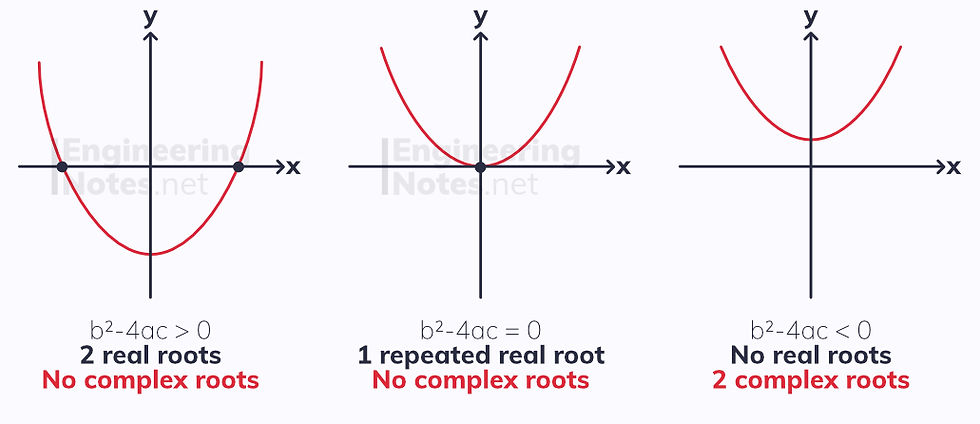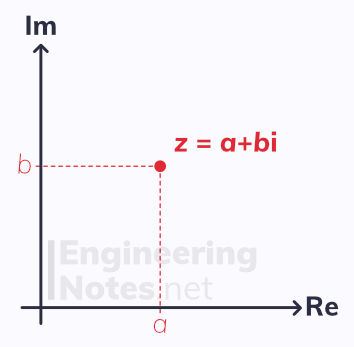top of page

#### Notes by Category University Engineering

Rate these notesNot a fanNot so goodGoodVery goodBrillRate these notes

# Complex Numbers, Argand Diagrams & Loci

We know from single maths that if the discriminant of a quadratic equation is positive, it has two solutions, if the discriminant = 0, there is one repeated root, and if it is negative there are no roots.

Wrong.

If the discriminant is negative, the quadratic has two imaginary roots, given in terms of i, the square root of -1:

i = √-1

A complex number is a sum of a real and an imaginary number, given in the form a + b i, where a is the real number and b the coefficient of i. Often, complex numbers are represented by the letter z.

### Adding and Subtracting Complex Numbers

To add or subtract complex numbers, treat the real and imaginary terms separately:

(a+b i) + (c+d i) = (a+c) + (b+d)i

A few examples:

• 3 + (2+4i) = (3+2) + (4i) = 5+4i

• (1+2i) - 4i = (1) + (2-4)i = 1-2i

• (2-2i) + (6+i) = (2+6) + (-2+1)i = 8-i

### Multiplying Complex Numbers

To multiply an imaginary number by a real constant, just expand the brackets:

k(a+b i) = ka+kb i

For example:

• 3(2+4i) = 3(2) + 3(4i) = 6+12i

To multiply two imaginary numbers, expand like any quadratic, using the fact that:

i² = -1

For example:

• (2+3i)(3+4i) = 6 + 8i +9i +12i² = 6 + 17i - 12 = -6+17i

### Complex Conjugation

Just like conjugate pairs when rationalising a denominator, the conjugate of a complex number has the opposite sign:

For any complex number z = a +bi, the conjugate is given as z* = a -bi

The complex conjugate is given an asterisk.

For any complex number z, the product of z and z* is a real number

For example, if z = 4+5i:

• z* = 4-5i

• zz* = (4+5i)(4-5i) = 16 + 20i - 20i -25i² = 16 + 25 = 41

## Solving Complex Equations

Complex roots always come in conjugate pairs. Therefore, if the discriminant of a quadratic is negative, if z is one of the roots, z* will be the other root.• For example, the roots of the equation x² - 6x + 13 = 0 are x = (3+2i) and x = (3-2i)

Often, equations with complex roots will use z instead of x.

The same rule applies to cubic and quartic graphs:• If a cubic graph has just one real root, it will have two complex roots, z and z*

• If a quartic graph has two real roots or one real repeated root, then it will also have two complex roots, z and z*

• If a quartic graph has no real roots, it will have two complex conjugate pairs as roots: z, z*, w and w*

All cubic graphs must have a total of three roots, real or complex
All quartic graphs must have a total of four roots, real or complex

## Argand Diagrams

Complex numbers can be represented graphically on an Argand diagram. The x-axis is the real axis, Re, and the y-axis is the imaginary axis, Im. To plot a complex number, treat it as a coordinate:The complex number z = (a + bi) has coordinates (a, b) on an Argand diagram

This can be represented as a position vector, at an angle, θ, to the positive real axis (generally in radians):Like any vector, the modulus (the magnitude) can be found using Pythagoras' theorem:

|z| = √(a²+b²)

and tan of the angle is given as:

tan(θ) = b/a

## Modulus-Argument FormThe magnitude of the position vector for a complex number, r, is known as the modulus, and the angle it makes with the positive real axis, arg z, is called the argument. Therefore, complex numbers can also be expressed in modulus-argument form:

z = r(cos(θ) + i sin(θ))
• r = |z|

• arg z = θ

### Multiplying & Dividing Mod-Arg## Loci

A locus of points is a collection of points that can be anywhere along a particular line. These can be represented with complex numbers on an Argand diagram.The distance between two complex numbers, z₁ and z₂, is given as |z₂ - z₁|

If, instead of defining both complex numbers, you just define one and give one general complex number, you get the locus of a circle:|z - z₁| = r is a circle with centre z₁ and radius r

This can also be given as |z - (a+bi)| = r, where r is the radius and (a, b) the centre.

You can find the locus of points for a perpendicular bisector of a line segment between two points:The perpendicular bisector of a line segment between two complex numbers, z₁ and z₂, is given as |z - z₁| = |z - z₂|

Using the argument of a complex number, z₁, we can express the locus of points for a half line. This is a line from, but not including, the fixed point z₁ at an angle of arg(z-z₁):arg(z-z₁) = θ is a half line from but not including the point z₁ at an angle of θ to the horizontal.

### Regions

Loci can be used to represent regions on an Argand diagram:## Exponential Form

Complex numbers can also be expressed in exponential form, z = r e^iθ, using Euler's relation:Euler's relation is derived from the Maclaurin series expansions of sin(θ), cos(θ) and eˣ. See Further Maths Notes Sheet on Series.

This leads to the exponential form for a complex number, z:• Where r = |z| and arg z